Get instant live expert help with Excel or Google Sheets“My Excelchat expert helped me in less than 20 minutes, saving me what would have been 5 hours of work!”

#### Post your problem and you’ll get Expert help in seconds.

Your message must be at least 40 characters
Our professional Expert are available now. Your privacy is guaranteed.

# Excel BIN2HEX Function

Binary numbers are commonly applied in computer technology. However, more people prefer to use hexadecimals in mathematics, computations and computer programming because it is easier to understand.

The table below shows the conversion of the decimal numbers 1 to 15 to binary and hexadecimals.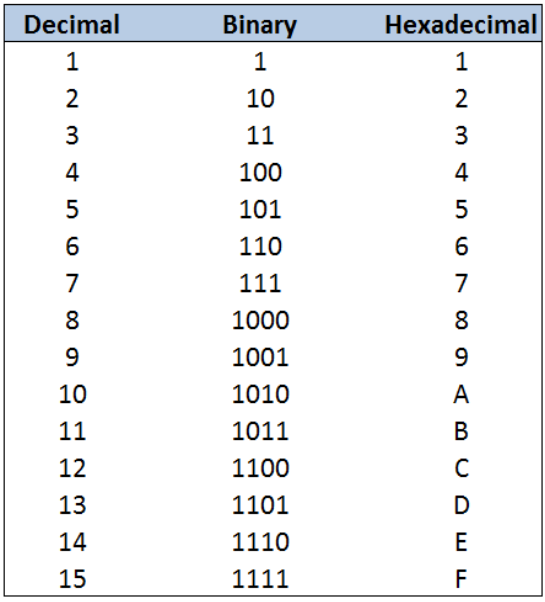Figure 1. Decimal numbers 1 to 15 converted to binary and hexadecimals

## BIN2HEX Function in Excel

BIN2HEX function converts a binary number to hexadecimal.

A binary number (BIN) is expressed in the base 2 numeral system, using only the digits 1 and 0; while a hexadecimal (HEX) is in the base 16 numeral system, using the numbers 0 to 9 and the letters A to F.

### Syntax

`=BIN2HEX(number, [places])`

Where

• number: binary number we want to convert to hexadecimal; maximum of 10 digits only
• places: optional; the number of characters to use in the hexadecimal; if omitted, BIN2HEX only uses the minimum number of necessary characters

## Setting up the Data

Below table shows some binary numbers to be converted to hexadecimal.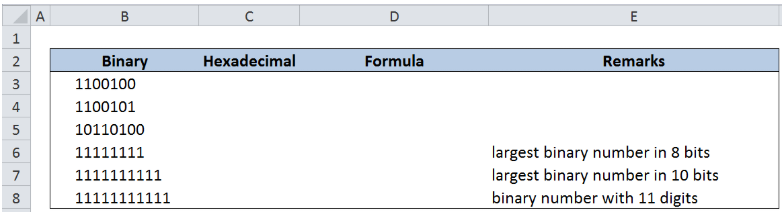Figure 2. Sample binary numbers for conversion to hexadecimal

Conversion of a binary number to hexadecimal in Excel in pretty straightforward.

In cell C3, enter the formula:

`=BIN2HEX(B3)`

The binary number 1100100 is converted to its hexadecimal value of 64.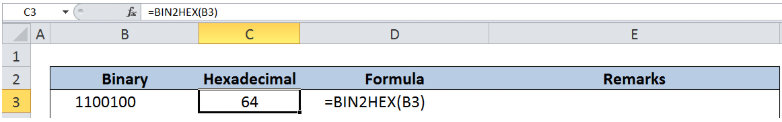Figure 3. Entering the formula for BIN2HEX

## Specifying the number of places

In cell C4, enter the formula

`=BIN2HEX(B4,3)`

The value for the argument “places” is 3, so Excel adds a zero “0” before the value 65 to return a three-digit hexadecimal “065”.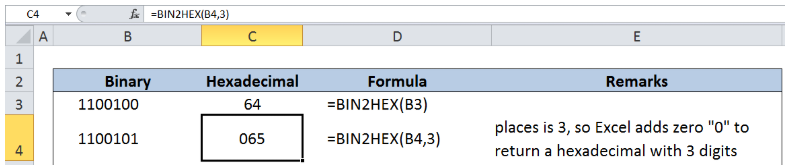Figure 4. Entering the BIN2HEX formula with value for “places”

Below table shows the conversion of the largest 8-bit and 10-bit binary numbers to hexadecimal.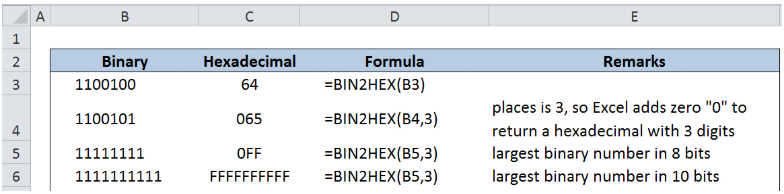Figure 5. More examples using BIN2HEX function

## Error Values in BIN2HEX

Below are the common reasons for the BIN2HEX function to return error values:

• If the binary number is not a valid binary number, BIN2HEX returns the #NUM! error value.
• Example: 101a converted to hexadecimal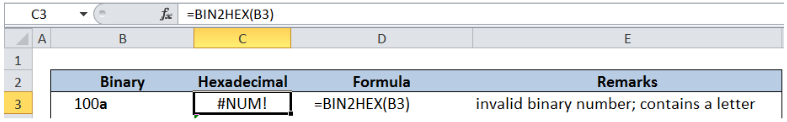Figure 6. BIN2HEX error with an invalid binary number

• If the binary number contains more than 10 characters (10 bits).
• Example: 11111111111 converted to hexadecimal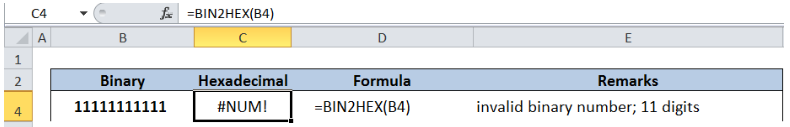Figure 7. BIN2HEX error with an 11-digit binary number

• Invalid value for places.
• Example: 11 as value for places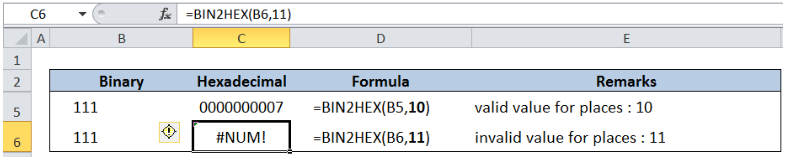Figure 8. BIN2HEX error with value for places > 10

• If places is not an integer.
• If places is nonnumeric, BIN2HEX returns the #NAME? error value.
• If places is negative, BIN2HEX returns the #NUM! error value.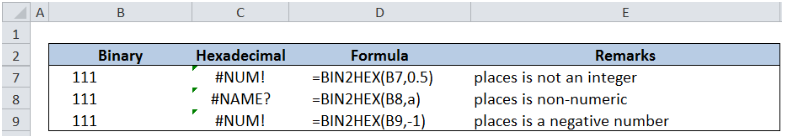Figure 9. BIN2HEX errors with invalid values for places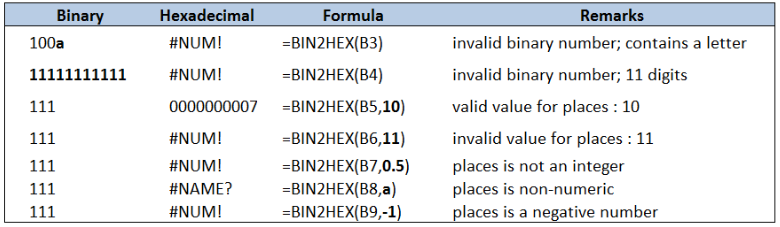Figure 10. Common errors with BIN2HEX function

Most of the time, the problem you will need to solve will be more complex than a simple application of a formula or function. If you want to save hours of research and frustration, try our live Excelchat service! Our Excel Experts are available 24/7 to answer any Excel question you may have. We guarantee a connection within 30 seconds and a customized solution within 20 minutes.